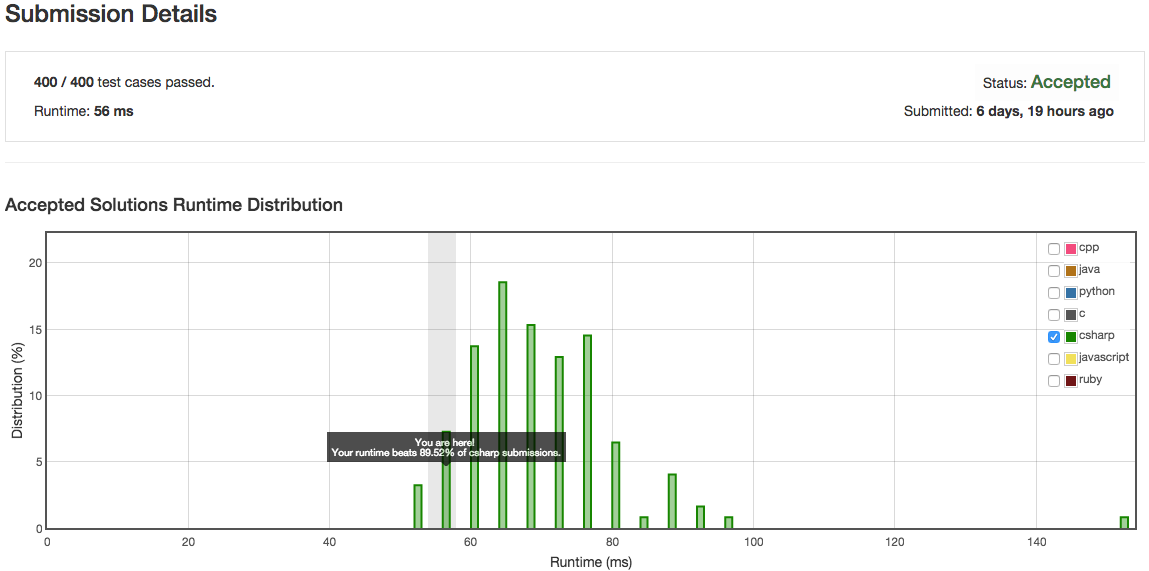LeetCode 的 Happy Number 題目如下：

Write an algorithm to determine if a number is “happy”.

A happy number is a number defined by the following process: Starting with any positive integer, replace the number by the sum of the squares of its digits, and repeat the process until the number equals 1 (where it will stay), or it loops endlessly in a cycle which does not include 1. Those numbers for which this process ends in 1 are happy numbers.

Example: 19 is a happy number

12 + 92 = 82
82 + 22 = 68
62 + 82 = 100
12 + 02 + 02 = 1

Happy number 為一個正整數，將數值的每個位數平方後相加，反覆這樣的邏輯，最後的數值會為 1。

1^2 + 9^2 = 82
8^2 + 2^2 = 68
6^2 + 8^2 = 100
1^2 + 0^2 + 0^2 = 1